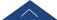﻿ 20220528 A Metric Geometry Day-湖南大学数学学院

# 20220528 A Metric Geometry Day

A Metric Geometry Day

Sponsored by National Natural Science Foundation of China (#12001179) and Hunan University, a mini workshop on Metric Geometry will take place in May 28, 2022, at Tencent Metting ID: 785 924 490.

Schledule of Events.

Morning Section: 8:30-11:30

Time: 8:30-9:30, HostMeng ZHU

Specker: Lina CHEN

Title: Almost maximal volume entropy rigidity for integral Ricci curvature in the non-collapsing case

Abstract: In this note we will show the almost maximal volume entropy rigidity for manifolds with lower integral Ricci curvature bound in the non-collapsing case: given $n,d,p>\frac{n}{2}$, there exist $\delta(n,d,p), \epsilon(n,d,p)>0$, such that for $\delta<\delta(n,d,p)$, $\epsilon<\epsilon(n,d,p)$, if a compact $n$-manifold $M$ satisfies that the integral Ricci curvature has lower bound $\bar k(-1,p)\leq \delta$, the diameter $\op{diam}(M)\leq d$ and volume entropy $h(M)\geq n-1-\epsilon$, then the universal cover of $M$ is Gromov-Hausdorff close to a hyperbolic space form $\Bbb H^k$, $k\leq n$; If in addition the volume of $M$, $\volume(M)\geq v>0$, then $M$ is diffeomorphic and Gromov-Hausdorff close to a hyperbolic manifold where $\delta, \epsilon$ also depends on $v$.

Time: 9:30-10:30, HostChengjie YU

Specker: Zhilang ZHANG

Abstract: In this talk, we will discuss some structure problems related to collapsed manifolds with boundary, where we assume a lower sectional curvature bound, two side bounds on the second fundamental forms of boundaries and upper diameter bound. Our main concern is the case when inradii of manifolds converge to zero. This is a typical case of collapsing manifolds with boundary. We determine the limit spaces of inradius collapsed manifolds as Alexandrov spaces with curvature uniformly bounded below. When the limit space has codimension one, we completely determine the topology of inradius collapsed manifold in terms of singular I-bundles. General inradius collapse to almost regular spaces are also  characterized. This talk is based on some recent joint work with Prof. Takao Yamaguchi.

Time: 10:30-11:30, HostXiantao HUANG

Specker: Yin JIANG

Title: Non-existence of concave functions on certain metric spaces

Abstract: In 1974, Yau proved that: there is no non-trivial continuous concave function on a complete manifold with finite volume. We will prove analogue theorems for several metric spaces.

Afternoon Section: 14:30-17:30

Free discussions.

Contact: Yashan ZHANG, yashanzh@hnu.edu.cn